# How to calculate cost per unit in Microsoft Excel

In this tutorial we will learn how to calculate cost per unit in Microsoft Excel. Calculating cost per unit is a very common task in Excel. It is calculated by dividing the total cost of production by the total number of units produced. Calculating cost per unit accurately is essential for businesses to make informed decisions about pricing, production, and profitability. Excel provides a user-friendly platform for performing complex calculations, making it an ideal tool for calculating cost per unit.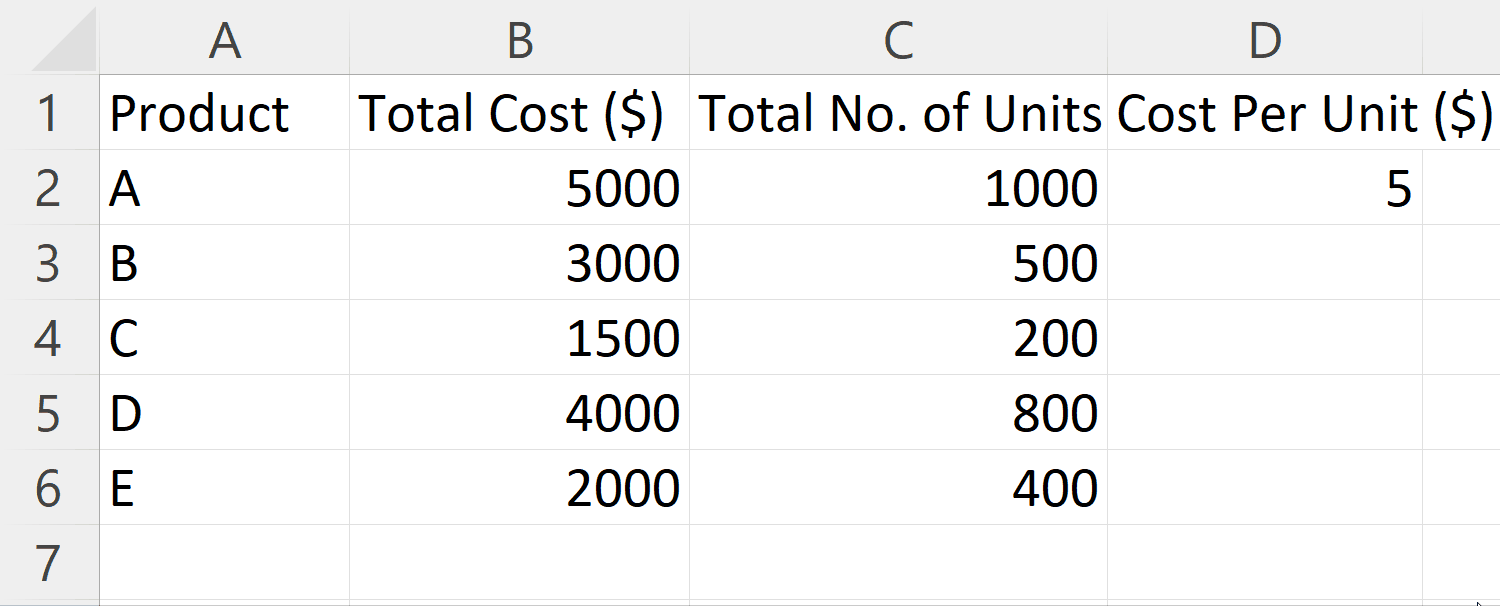Calculating cost per unit in Excel is useful for businesses to track and monitor their expenses and profitability. By calculating cost per unit, businesses can make informed decisions about pricing their products and services, determining the profitability of different products, and identifying areas where they can cut costs to improve profitability.

### Step 1 – Organize the Data– In order to determine the cost per unit accurately, it is necessary to organize the data in a specific format.
– This involves creating a table with three columns: Products, Total Units, and Total Cost.

### Step 2 – Select a Blank Cell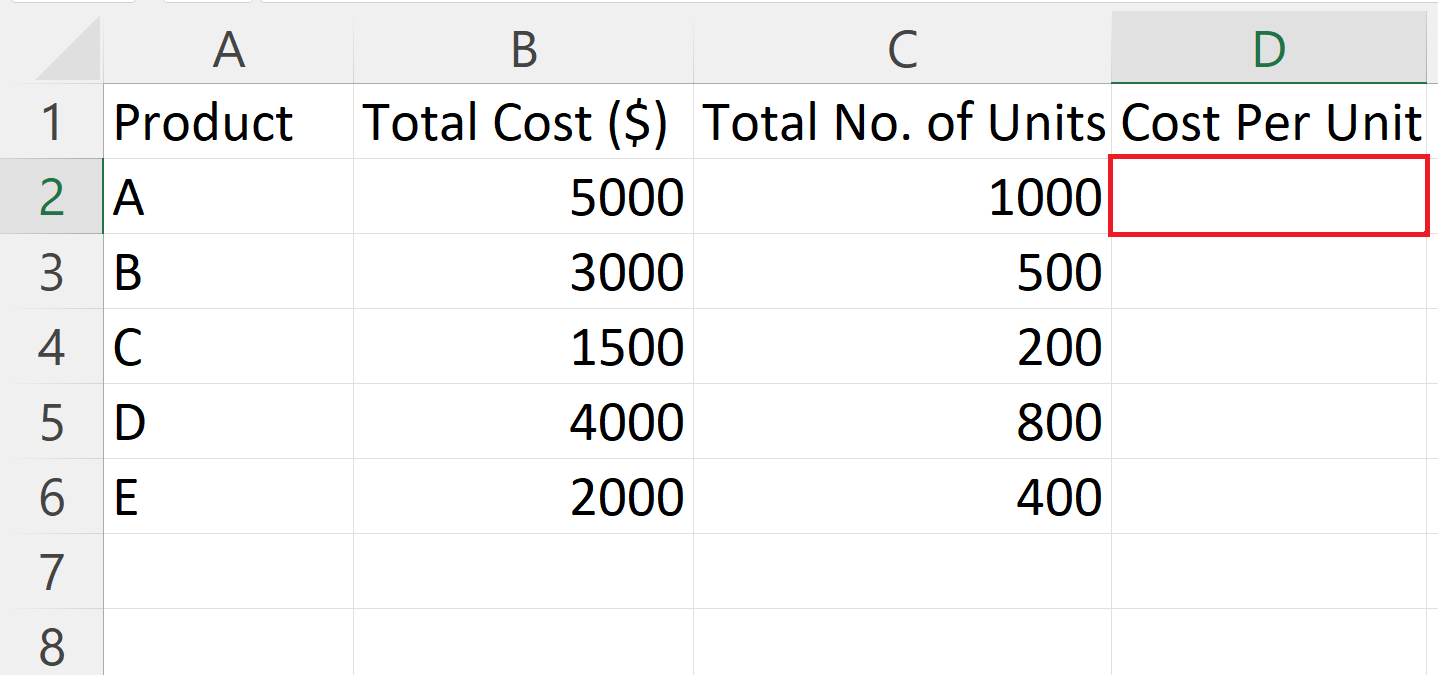– Select a blank targeted cell where you want to calculate the cost per unit.

### Step 3 – Place an Equals Sign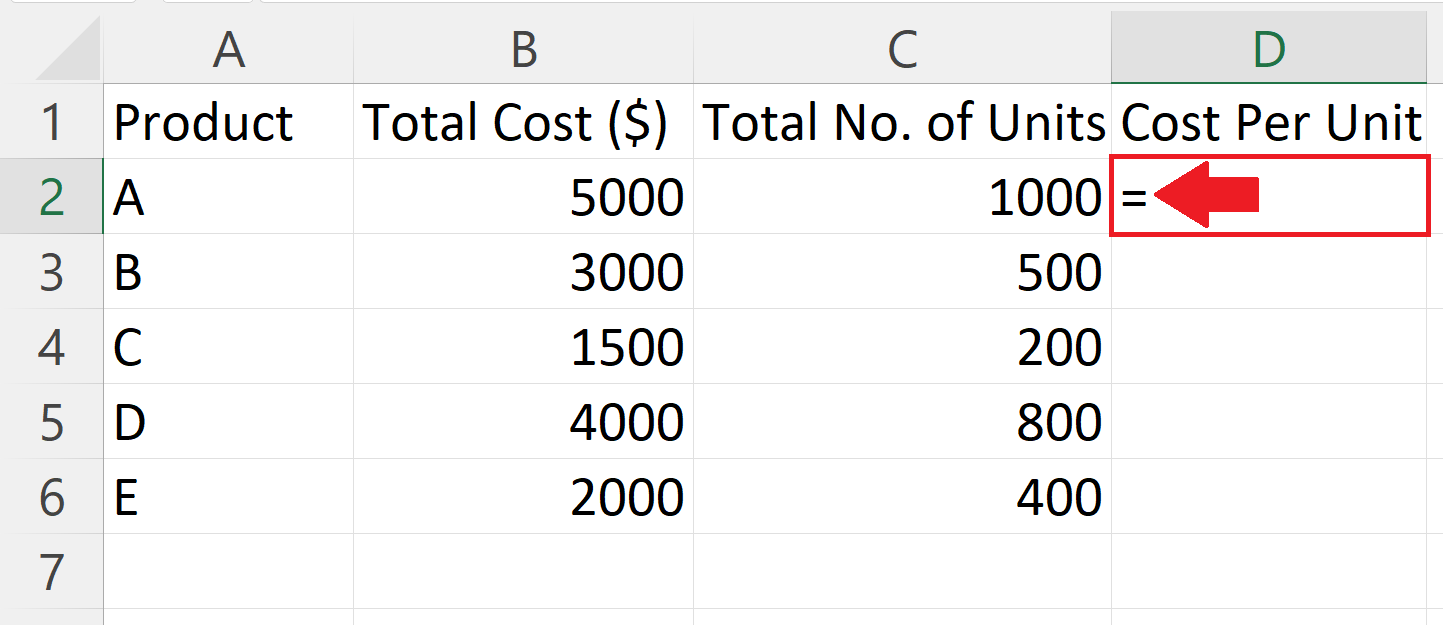### Step 4 – Enter the Formula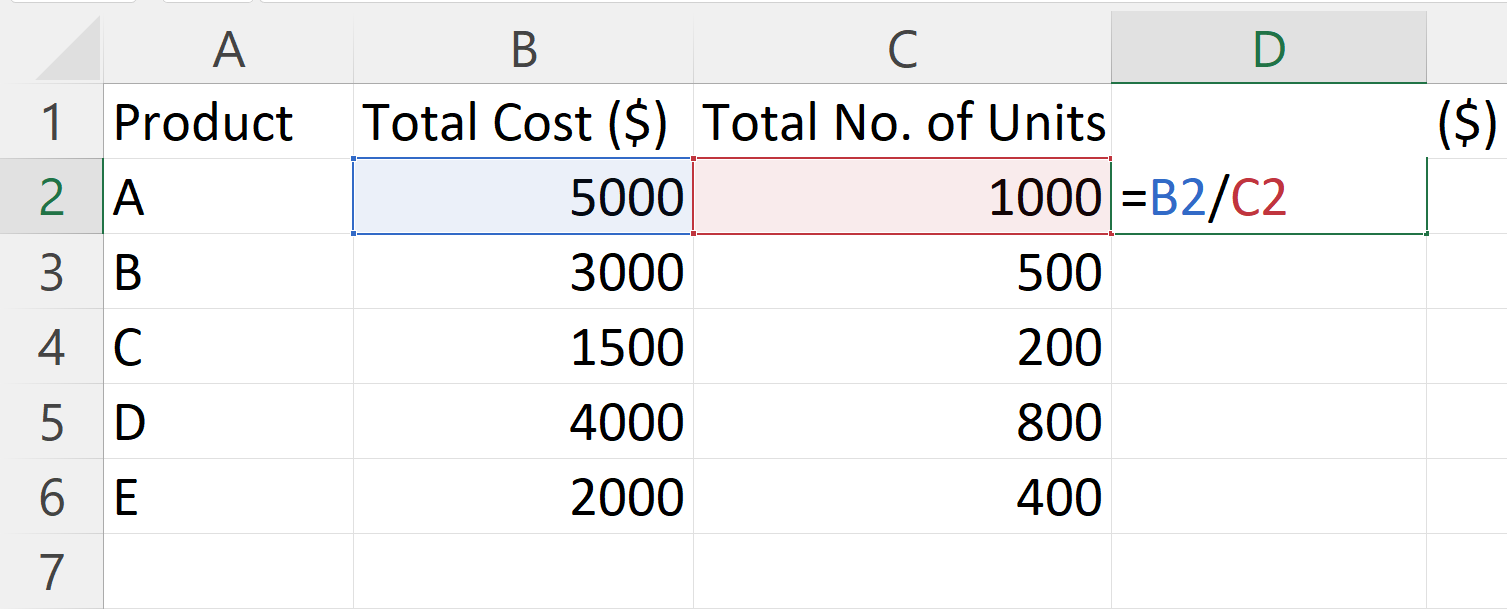– Enter the formula to calculate the cost per unit.
– We will use the following formula:
C2/B2
– Where C2 is the Total Cost and B2 is the Number of Total Units.

### Step 5 – Press the Enter Key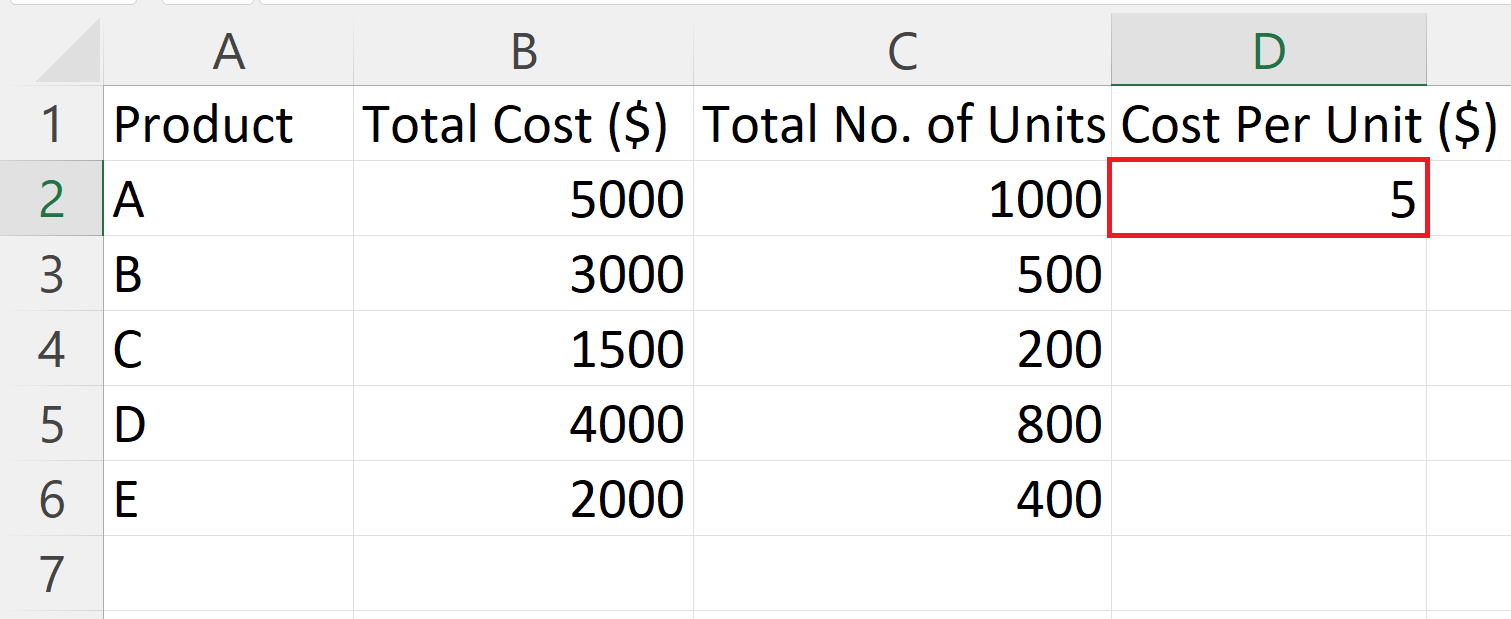– Press the Enter key to calculate the cost per unit.

### Step 6 – Apply the Formula on All the Rows– Apply the formula on all the rows containing products to calculate cost per unit for each.